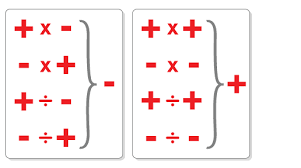# Negative numbersWorking with negative numbers can be confusing, but a few simple rules can help you add, subtract, multiply and divide successfully:

If the operator and the sign are the same, then you must add the number, eg 4 – -3 = 4 + 3 = 7 or 4 + +3 = 7.

If the operator and the sign are different, then you must subtract the number, eg 4 + -3 = 4 – 3 = 1 or 4 – +3 = 1.

Here are a few practice questions:

1. 14 – -3
2. -4 + -7
3. 21 – +8
4. -8 + -7
5. 6 – -4

Multiplication and division

If the signs of the two numbers are the same, then you must make the answer positive, eg -4 x -3 = 4 x 3 = 12 or +4 x +3 = 4 x 3 = 12.

If the signs of the two numbers are different, then you must make the answer negative, eg -4 x +3 = -12 or +4 x -3 = -12.

Here are a few practice questions:

1. 1 × -3
2. -8 ÷ -4
3. 2 × -7
4. -14 ÷ -7
5. 6 × -4